# Inheritance in Python

## Inheritance in Python

In this article, I am going to discuss Inheritance in Python with examples. Please read our previous article where we discussed Types of Class Methods in Python. As part of this article, we are going to discuss the following pointers which are related to Inheritance in Python.

1. What is Inheritance?
3. Implementation of Inheritance in Python
4. Single Inheritance in Python
5. Multilevel Inheritance
6. Multiple Inheritance
7. What if both parent classes have a method with the same name?
8. Constructors in Inheritance
9. Can we call super class constructor from child class constructor?
##### What is Inheritance in Python:

Inheritance, in general, is the passing of properties to someone. In programming languages, the concept of inheritance comes with classes.

1. Creating new classes from already existing classes is called inheritance.
2. The existing class is called a super class or base class or parent class.
3. The new class is called a subclass or derived class or child class.
4. Inheritance allows sub classes to inherit the variables, methods and constructors of their super class.
1. The main advantage of inheritance is code re-usability.
2. Time taken for application development will be less.
3. Redundancy (repetition) of the code can be reduced.
##### Implementation of Inheritance in Python:

While declaring subclass, we need to pass super class name into subclass’s parenthesis

Program: Implementing Inheritance (demo1.py)

```class One:
def m1(self):
print("Parent class m1 method")
class Two(One):
def m2(self):
print("Child class m2 method")
c = Two()
c.m1()
c.m2()```

Output: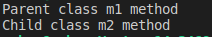##### Types of Inheritance in Python:

There are there types of inheritance, they are:

1. Single inheritance
2. Multilevel inheritance
3. Multiple inheritance
##### SINGLE INHERITANCE in PYTHON:

Creating a subclass or child class from a single superclass/parent class is called single inheritance. Diagrammatic representation of Python Single Inheritance is given below.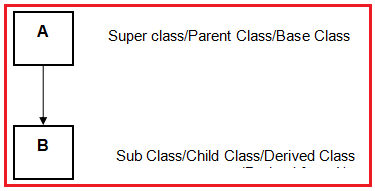Program: Single Inheritance (demo2.py)

```class A:
def m1(self):
print("A class m1 Method")
class B(A):
def m2(self):
print("Child B is derived from A class: m2 Method")
obj=B()
obj.m1()
obj.m2()```

Output: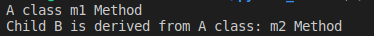##### MULTILEVEL INHERITANCE in PYTHON:

If a class is derived from another derived class then it is called multi level inheritance. Diagrammatic representation of Python Multilevel Inheritance is given below.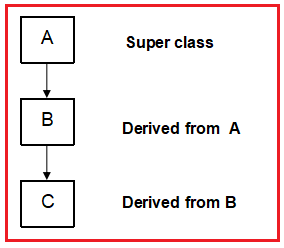Program: Multilevel inheritance (demo3.py)

```class A:
def m1(self):
print("Parent class A: m1 Method")
class B(A):
def m2(self):
print("Child class B derived from A: m2 Method")
class C(B):
def m3(self):
print("Child class C derived from B: m3 Method")
obj=C()
obj.m1()
obj.m2()
obj.m3()```

Output: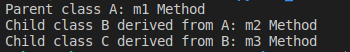##### MULTIPLE INHERITANCE in PYTHON:

If a child class is derived from multiple superclasses then it is called multiple inheritance. Diagrammatic representation of Multiple Inheritance is given below.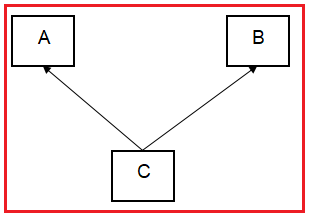Program: Multiple Inheritance (demo4.py)

```class P1:
def m1(self):
print("Parent1 Method")
class P2:
def m2(self):
print("Parent2 Method")
class C(P1, P2):
def m3(self):
print("Child Method")
c=C()
c.m1()
c.m2()
c.m3()```

Output: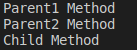##### What if both parent classes have a method with the same name?

There may be a chance that two parent classes can contain methods which are having the same method name in both classes. Syntactically this is valid.

Program: Parent classes having same method names (demo5.py)

```class P1:
def m1(self):
print("Parent1 Method")
class P2:
def m1(self):
print("Parent2 Method")
class C(P1, P2):
def m2(self):
print("Child Method")
c=C()
c.m2()```

Output: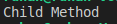##### In the above scenario, which method will child class inherit?

The answer for this depends on the order of inheritance of parent classes in the child class.

1. class C(P1, P2): ===>P1”s class method will be considered
2. class C(P2, P1): ===>P2”s class method will be considered

Program: demo5.py contd

```class P1:
def m1(self):
print("Parent1 Method")
class P2:
def m1(self):
print("Parent2 Method")
class C(P1, P2):
def m2(self):
print("Child Method")
c=C()
c.m2()
c.m1()```

Output: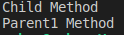Program: Other scenario of demo5.py

```class P1:
def m1(self):
print("Parent1 Method")
class P2:
def m1(self):
print("Parent2 Method")
class C(P2, P1):
def m2(self):
print("Child Method")
c=C()
c.m2()
c.m1()```

Output: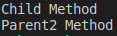##### CONSTRUCTORS in INHERITANCE

By default, the super class‟s constructor will be available to the subclass.

Program:demo6.py

```class A:
def __init__(self):
print("super class A constructor")

class B(A):
def m1():
print("Child Class B: m1 method from B")

b=B()```

Output: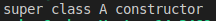##### If child class and super class both have constructors, then?

If child class and super class both have constructors, if you create an object to child class then child class constructor will be executed. While creating object for a class, that class’s constructor is first priority.

Program: demo7.py

```class A:
def __init__(self):
print("super class A constructor")

class B(A):
def __init__(self):
print("Child class B constructor")

b=B()```

Output: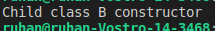##### Can we call super class constructor from child class constructor?

Yes, we can call super class constructor from child class constructor by using super() function. super() is a predefined function which is useful to call the superclass constructors, variables and methods from the child class.

Program: demo8.py

```class A:
def __init__(self):
print("super class A constructor")

class B(A):
def __init__(self):
print("Child class B constructor")
super().__init__()

b=B()```

Output: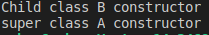1.